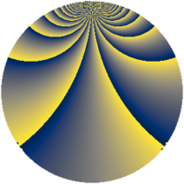# Properties

 Label 588.2.xLevel $588$ Weight $2$ Character orbit 588.x Rep. character $\chi_{588}(55,\cdot)$ Character field $\Q(\zeta_{14})$ Dimension $336$ Newform subspaces $2$ Sturm bound $224$ Trace bound $3$

# Related objects

## Defining parameters

 Level: $$N$$ $$=$$ $$588 = 2^{2} \cdot 3 \cdot 7^{2}$$ Weight: $$k$$ $$=$$ $$2$$ Character orbit: $$[\chi]$$ $$=$$ 588.x (of order $$14$$ and degree $$6$$) Character conductor: $$\operatorname{cond}(\chi)$$ $$=$$ $$196$$ Character field: $$\Q(\zeta_{14})$$ Newform subspaces: $$2$$ Sturm bound: $$224$$ Trace bound: $$3$$ Distinguishing $$T_p$$: $$11$$

## Dimensions

The following table gives the dimensions of various subspaces of $$M_{2}(588, [\chi])$$.

Total New Old
Modular forms 696 336 360
Cusp forms 648 336 312
Eisenstein series 48 0 48

## Trace form

 $$336 q + 12 q^{8} - 56 q^{9} + O(q^{10})$$ $$336 q + 12 q^{8} - 56 q^{9} + 2 q^{14} + 16 q^{16} + 4 q^{21} - 12 q^{22} - 42 q^{24} + 64 q^{25} - 60 q^{28} - 16 q^{30} - 20 q^{32} - 112 q^{34} - 20 q^{37} + 56 q^{40} + 16 q^{42} + 40 q^{44} - 16 q^{49} + 228 q^{50} - 16 q^{53} + 22 q^{56} + 24 q^{57} - 12 q^{58} + 20 q^{60} - 28 q^{61} - 16 q^{65} + 78 q^{70} - 30 q^{72} + 44 q^{74} - 42 q^{76} + 16 q^{77} + 12 q^{78} - 56 q^{81} - 16 q^{84} - 48 q^{85} - 40 q^{86} - 148 q^{88} + 14 q^{92} - 8 q^{93} - 42 q^{94} - 268 q^{98} + O(q^{100})$$

## Decomposition of $$S_{2}^{\mathrm{new}}(588, [\chi])$$ into newform subspaces

Label Dim $A$ Field CM Traces $q$-expansion
$a_{2}$ $a_{3}$ $a_{5}$ $a_{7}$
588.2.x.a $168$ $4.695$ None $$0$$ $$-28$$ $$0$$ $$2$$
588.2.x.b $168$ $4.695$ None $$0$$ $$28$$ $$0$$ $$-2$$

## Decomposition of $$S_{2}^{\mathrm{old}}(588, [\chi])$$ into lower level spaces

$$S_{2}^{\mathrm{old}}(588, [\chi]) \cong$$ $$S_{2}^{\mathrm{new}}(196, [\chi])$$$$^{\oplus 2}$$